Showing first {{hits.length}} results of {{hits_total}} for {{searchQueryText}}{{hits.length}} results for {{searchQueryText}}

검색 결과 없음

The package CircuiTikz provides a set of macros for naturally typesetting electrical and electronic networks. This article explains basic usage of this package. Contents 1 Introduction 2 A working example 3 List of nodes 4 Further reading Introduction CircuiTikz includes several nodes that can be used with standard tikz syntax. \documentclass{article} \usepackage[utf8]{inputenc} \usepackage[english]{babel} \usepackage{circuitikz} \begin{document} \begin{center} \begin{circuitikz} \draw (0,0) to[ variable cute inductor ] (2,0); \end{circuitikz} \end{center} \end{document}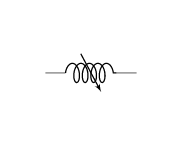To use the package it must be imported with \usepackage{circuitikz} in the preamble. Then the environment circuitikz is used to typeset the diagram with tikz syntax. In the example a node called variable cute inductor is used.   Open an example of the circuitikz package in Overleaf A working example As mentioned before, to draw electrical network diagrams you should use tikz syntax, the examples even work if the environment tikzpicture is used instead of circuitikz; below a more complex example is presented. \begin{center} \begin{circuitikz}[american voltages] \draw (0,0) to [short, *-] (6,0) to [V, l_=$\mathrm{j}{\omega}_m \underline{\psi}^s_R$] (6,2) to [R, l_=$R_R$] (6,4) to [short, i_=$\underline{i}^s_R$] (5,4) (0,0) to [open, v^>=$\underline{u}^s_s$] (0,4) to [short, *- ,i=$\underline{i}^s_s$] (1,4) to [R, l=$R_s$] (3,4) to [L, l=$L_{\sigma}$] (5,4) to [short, i_=$\underline{i}^s_M$] (5,3) to [L, l_=$L_M$] (5,0); \end{circuitikz} \end{center}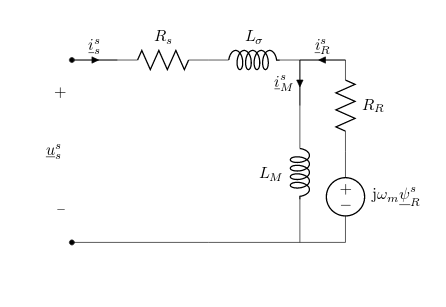The nodes short, V, R and L are presented here, but there a lot more. Some of them are presented in the next section.   Open an example of the circuitikz package in Overleaf List of nodes Below most of the elements provided by CircuiTikz are listed: Monopoles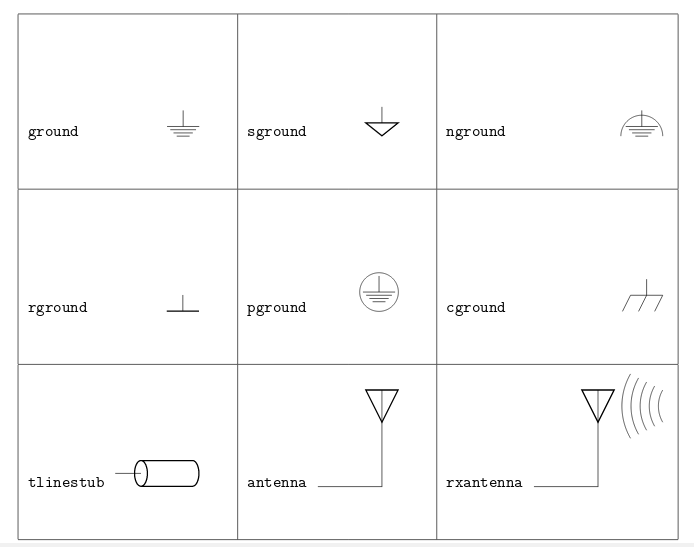Bipoles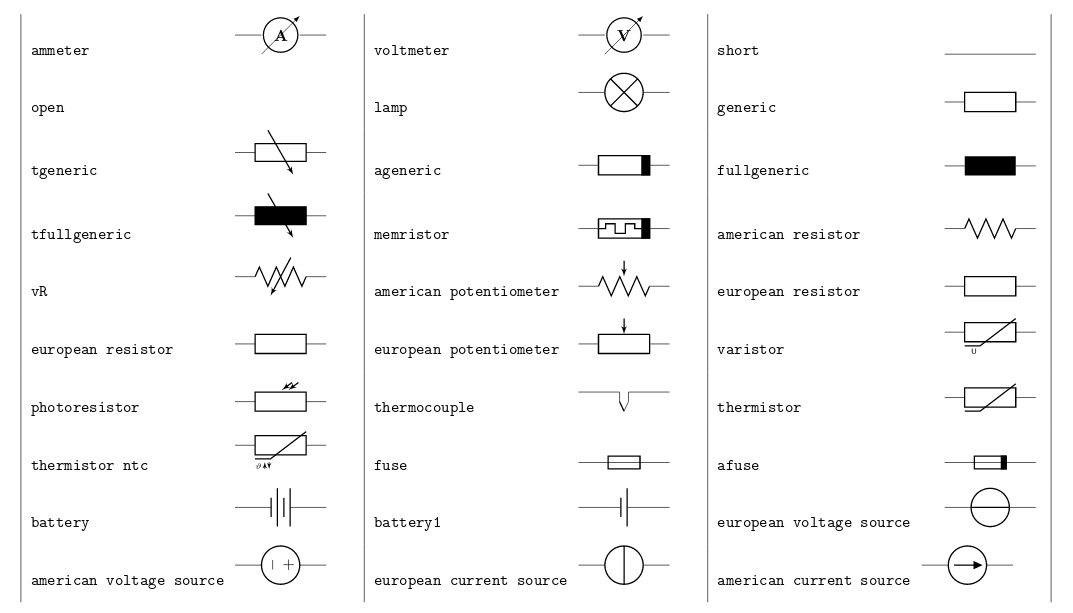Diodes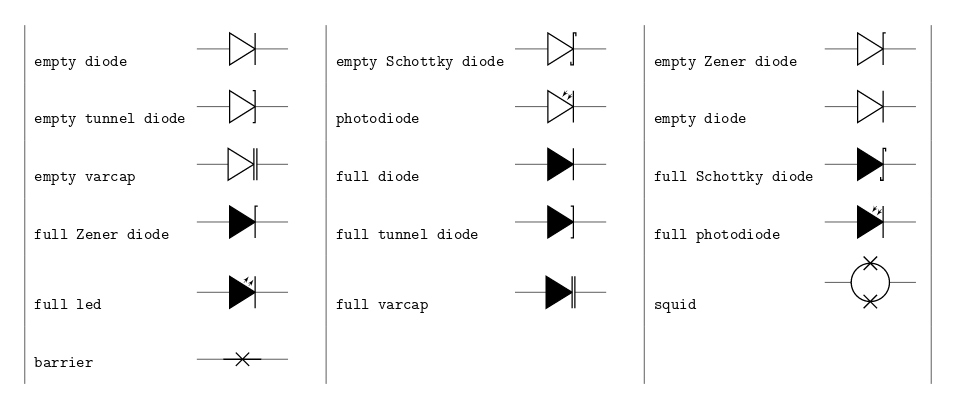dynamical bipoles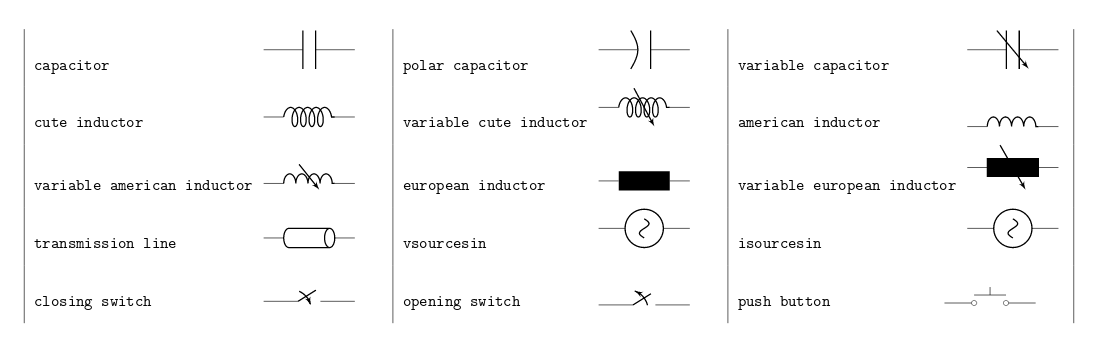Open an example of the circuitikz package in Overleaf Further reading For more information see: The TikZ guide Inserting Images Positioning images and tables Lists of tables and figures Using colours in LaTeX Lengths in LaTeX The circuitikz package documentation